# Hyperbolic geometry

﻿
Hyperbolic geometry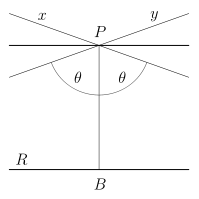Lines through a given point P and asymptotic to line R.A triangle immersed in a saddle-shape plane (a hyperbolic paraboloid), as well as two diverging ultraparallel lines.

In mathematics, hyperbolic geometry (also called Lobachevskian geometry or Bolyai-Lobachevskian geometry) is a non-Euclidean geometry, meaning that the parallel postulate of Euclidean geometry is replaced. The parallel postulate in Euclidean geometry is equivalent to the statement that, in two dimensional space, for any given line R and point P not on R, there is exactly one line through P that does not intersect R; i.e., that is parallel to R. In hyperbolic geometry there are at least two distinct lines through P which do not intersect R, so the parallel postulate is false. Models have been constructed within Euclidean geometry that obey the axioms of hyperbolic geometry, thus proving that the parallel postulate is independent of the other postulates of Euclid.

Because there is no precise hyperbolic analogue to Euclidean parallel lines, the hyperbolic use of parallel and related terms varies among writers. In this article, the two limiting lines are called asymptotic and lines sharing a common perpendicular are called ultraparallel; the simple word parallel may apply to both.

A characteristic property of hyperbolic geometry is that the angles of a triangle add to less than a straight angle. In the limit as the vertices go to infinity, there are even ideal hyperbolic triangles in which all three angles are 0°.

## Non-intersecting lines

An interesting property of hyperbolic geometry follows from the occurrence of more than one line parallel to R through a point P, not on R: there are two classes of non-intersecting lines. Let B be the point on R such that the line PB is perpendicular to R. Consider the line x through P such that x does not intersect R, and the angle θ between PB and x counterclockwise from PB is as small as possible; i.e., any smaller angle will force the line to intersect R. This is called an asymptotic line in hyperbolic geometry. Symmetrically, the line y that forms the same angle θ between PB and itself but clockwise from PB will also be asymptotic. x and y are the only two lines asymptotic to R through P. All other lines through P not intersecting R, with angles greater than θ with PB, are called ultraparallel (or disjointly parallel) to R. Notice that since there are an infinite number of possible angles between θ and 90°, and each one will determine two lines through P and disjointly parallel to R, there exist an infinite number of ultraparallel lines.

Thus we have this modified form of the parallel postulate: In hyperbolic geometry, given any line R, and point P not on R, there are exactly two lines through P which are asymptotic to R, and infinitely many lines through P ultraparallel to R.

The differences between these types of lines can also be looked at in the following way: the distance between asymptotic lines shrinks toward zero in one direction and grows without bound in the other; the distance between ultraparallel lines (eventually) increases in both directions. The ultraparallel theorem states that there is a unique line in the hyperbolic plane that is perpendicular to each of a given pair of ultraparallel lines.

In Euclidean geometry, the "angle of parallelism" is a constant; that is, any distance$\lVert BP \rVert$ between parallel lines yields an angle of parallelism equal to 90°. In hyperbolic geometry, the angle of parallelism varies with the Π(p) function. This function, described by Nikolai Ivanovich Lobachevsky, produces a unique angle of parallelism for each distance p =$\lVert BP \rVert$. As the distance gets shorter, Π(p) approaches 90°, whereas with increasing distance Π(p) approaches 0°. Thus, as distances get smaller, the hyperbolic plane behaves more and more like Euclidean geometry. Indeed, on small scales compared to$\frac{1}{\sqrt{-K}}$, where K is the (constant) Gaussian curvature of the plane, an observer would have a hard time determining whether the environment is Euclidean or hyperbolic.

## Triangles

Distances in the hyperbolic plane can be measured in terms of a unit of length$R = \frac{1}{\sqrt{-K}}$ , analogous to the radius of the sphere in spherical geometry. Using this unit of length a theorem in hyperbolic geometry can be stated which is analogous to the Pythagorean theorem. If a, b are the legs and c is the hypotenuse of a right triangle all measured in this unit then:$\cosh c=\cosh a\cosh b\,.$

The cosh function is a hyperbolic function which is an analog of the standard cosine function. All six of the standard trigonometric functions have hyperbolic analogs. In trigonometric relations involving the sides and angles of a hyperbolic triangle the hyperbolic functions are applied to the sides and the standard trigonometric functions are applied to the angles. For example the law of sines for hyperbolic triangles is:$\frac{\sin A}{\sinh a} = \frac{\sin B}{\sinh b} = \frac{\sin C}{\sinh c}.$

For more of these trigonometric relationships see hyperbolic triangles.

Unlike Euclidean triangles whose angles always add up to 180° or π radians the sum of the angles of a hyperbolic triangle is always strictly less than 180°. The difference is sometimes referred to as the defect. The area of a hyperbolic triangle is given by its defect multiplied by R² where$R = \frac{1}{\sqrt{-K}}$. As a consequence all hyperbolic triangles have an area which is less than R²π. The area of an ideal hyperbolic triangle is equal to this maximum.

As in spherical geometry the only similar triangles are congruent triangles.

## Circles, spheres and balls

In hyperbolic geometry the circumference of a circle of radius r is greater than 2πr. It is in fact equal to$2\pi R \sinh \frac{r}{R} \,.$

The area of the enclosed disk is$2\pi R^2 (\cosh \frac{r}{R} - 1) \,.$

The surface area of a sphere is$4\pi R^2 \sinh^2 \frac{r}{R} \,.$

The volume of the enclosed ball is$\pi R^3 \sinh \frac{2r}{R} - 2\pi R^2r \,.$

For the measure of an n-1 sphere in n dimensional space the corresponding expression is$\Omega_{n} R^{n-1} \sinh^{n-1} \frac{r}{R} \,$

where the full n dimensional solid angle is$\Omega_{n}=\frac{2\pi^{n/2}}{\Gamma \left (\frac{n}{2} \right )} \,$

using$\Gamma \,$ for the Gamma function.

The measure of the enclosed n ball is:$\Omega_{n} R^{n-1} \int_0^r \sinh^{n-1} \frac{r}{R}dr \,.$

## History

A number of geometers made attempts to prove the parallel postulate by assuming its negation and trying to derive a contradiction, including Proclus, Ibn al-Haytham (Alhacen), Omar Khayyám, Nasir al-Din al-Tusi, Witelo, Gersonides, Alfonso, and later Giovanni Gerolamo Saccheri, John Wallis, Johann Heinrich Lambert, and Legendre. Their attempts failed, but their efforts gave birth to hyperbolic geometry.

The theorems of Alhacen, Khayyam and al-Tusi on quadrilaterals, including the Ibn al-Haytham–Lambert quadrilateral and Khayyam–Saccheri quadrilateral, were the first theorems on hyperbolic geometry. Their works on hyperbolic geometry had a considerable influence on its development among later European geometers, including Witelo, Gersonides, Alfonso, John Wallis and Saccheri.

In the 18th century, Johann Heinrich Lambert introduced the hyperbolic functions and computed the area of a hyperbolic triangle.

In the nineteenth century, hyperbolic geometry was extensively explored by János Bolyai and Nikolai Ivanovich Lobachevsky, after whom it sometimes is named. Lobachevsky published in 1830, while Bolyai independently discovered it and published in 1832. Carl Friedrich Gauss also studied hyperbolic geometry, describing in a 1824 letter to Taurinus that he had constructed it, but did not publish his work. In 1868, Eugenio Beltrami provided models of it, and used this to prove that hyperbolic geometry was consistent if Euclidean geometry was.

The term "hyperbolic geometry" was introduced by Felix Klein in 1871.

For more history, see article on non-Euclidean geometry, and the references Coxeter and Milnor.

## Models of the hyperbolic plane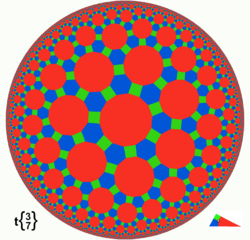Poincaré disc model of great rhombitruncated {3,7} tiling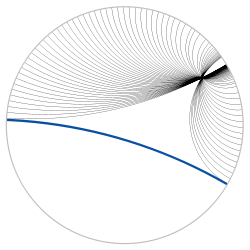Lines through a given point and asymptotic to a given line, illustrated in the Poincaré disc model

There are four models commonly used for hyperbolic geometry: the Klein model, the Poincaré disc model, the Poincaré half-plane model, and the Lorentz model, or hyperboloid model. These models define a real hyperbolic space which satisfies the axioms of a hyperbolic geometry. Despite their names, the first three mentioned above were introduced as models of hyperbolic space by Beltrami, not by Poincaré or Klein.

1. The Klein model, also known as the projective disc model and Beltrami-Klein model, uses the interior of a circle for the hyperbolic plane, and chords of the circle as lines.
2. The Poincaré disc model, also known as the conformal disc model, also employs the interior of a circle, but lines are represented by arcs of circles that are orthogonal to the boundary circle, plus diameters of the boundary circle.
3. The Poincaré half-plane model takes one-half of the Euclidean plane, as determined by a Euclidean line B, to be the hyperbolic plane (B itself is not included).
• Hyperbolic lines are then either half-circles orthogonal to B or rays perpendicular to B.
• Both Poincaré models preserve hyperbolic angles, and are thereby conformal. All isometries within these models are therefore Möbius transformations.
• The half-plane model is identical (at the limit) to the Poincaré disc model at the edge of the disc
4. The Lorentz model or hyperboloid model employs a 2-dimensional hyperboloid of revolution (of two sheets, but using one) embedded in 3-dimensional Minkowski space. This model is generally credited to Poincaré, but Reynolds (see below) says that Wilhelm Killing and Karl Weierstrass used this model from 1872.
• This model has direct application to special relativity, as Minkowski 3-space is a model for spacetime, suppressing one spatial dimension. One can take the hyperboloid to represent the events that various moving observers, radiating outward in a spatial plane from a single point, will reach in a fixed proper time. The hyperbolic distance between two points on the hyperboloid can then be identified with the relative rapidity between the two corresponding observers.

### Connection between the models

The four models essentially describe the same structure. The difference between them is that they represent different coordinate charts laid down on the same metric space, namely the hyperbolic space. The characteristic feature of the hyperbolic space itself is that it has a constant negative scalar curvature, which is indifferent to the coordinate chart used. The geodesics are similarly invariant: that is, geodesics map to geodesics under coordinate transformation. Hyperbolic geometry generally is introduced in terms of the geodesics and their intersections on the hyperbolic space.

Once we choose a coordinate chart (one of the "models"), we can always embed it in a Euclidean space of same dimension, but the embedding is clearly not isometric (since the scalar curvature of Euclidean space is 0). The hyperbolic space can be represented by infinitely many different charts; but the embeddings in Euclidean space due to these four specific charts show some interesting characteristics.

Since the four models describe the same metric space, each can be transformed into the other. See, for example, the Beltrami–Klein model's relation to the hyperboloid model, the Beltrami–Klein model's relation to the Poincaré disk model, and the Poincaré disk model's relation to the hyperboloid model.

## Visualizing hyperbolic geometry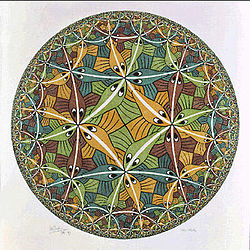M.C. Escher's Circle Limit III, 1959A collection of crocheted hyperbolic planes, in imitation of a coral reef, by the Institute For Figuring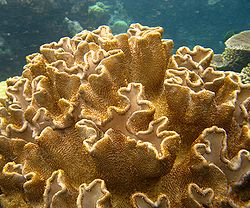A coral with similar geometry on the Great Barrier Reef

M. C. Escher's famous prints Circle Limit III and Circle Limit IV illustrate the conformal disc model quite well. The white lines in III are not quite geodesics (they are hypercycles), but are quite close to them. It is also possible to see quite plainly the negative curvature of the hyperbolic plane, through its effect on the sum of angles in triangles and squares.

For example, in Circle Limit III every vertex belongs to three triangles and three squares. In the Euclidean plane, their angles would sum to 450°; i.e., a circle and a quarter. From this we see that the sum of angles of a triangle in the hyperbolic plane must be smaller than 180°. Another visible property is exponential growth. In Circle Limit III, for example, one can see that the number of fishes within a distance of n from the center rises exponentially. The fishes have equal hyperbolic area, so the area of a ball of radius n must rise exponentially in n.

There are several ways to physically realize a hyperbolic plane (or approximation thereof). A particularly well-known paper model based on the pseudosphere is due to William Thurston. The art of crochet has been used to demonstrate hyperbolic planes with the first being made by Daina Taimina, whose book Crocheting Adventures with Hyperbolic Planes won the 2009 Bookseller/Diagram Prize for Oddest Title of the Year. In 2000, Keith Henderson demonstrated a quick-to-make paper model dubbed the "hyperbolic soccerball". Instructions on how to make a hyperbolic quilt, designed by Helaman Ferguson, has been made available by Jeff Weeks.

## Homogeneous structure

Hyperbolic space of dimension n is a special case of a Riemannian symmetric space of noncompact type, as it is isomorphic to the quotient$O(1,n)/(O(1) \times O(n))$.

The orthogonal group O(1,n) acts by norm-preserving transformations on Minkowski space R1,n, and it acts transitively on the two-sheet hyperboloid of norm 1 vectors. Timelike lines (i.e., those with positive-norm tangents) through the origin pass through antipodal points in the hyperboloid, so the space of such lines yields a model of hyperbolic n-space. The stabilizer of any particular line is isomorphic to the product of the orthogonal groups O(n) and O(1), where O(n) acts on the tangent space of a point in the hyperboloid, and O(1) reflects the line through the origin. Many of the elementary concepts in hyperbolic geometry can be described in linear algebraic terms: geodesic paths are described by intersections with planes through the origin, dihedral angles between hyperplanes can be described by inner products of normal vectors, and hyperbolic reflection groups can be given explicit matrix realizations.

In small dimensions, there are exceptional isomorphisms of Lie groups that yield additional ways to consider symmetries of hyperbolic spaces. For example, in dimension 2, the isomorphisms SO+(1,2) ≅ PSL(2,R) ≅ PSU(1,1) allow one to interpret the upper half plane model as the quotient SL(2,R)/SO(2) and the Poincaré disc model as the quotient SU(1,1)/U(1). In both cases, the symmetry groups act by fractional linear transformations, since both groups are the orientation-preserving stabilizers in PGL(2,C) of the respective subspaces of the Riemann sphere. The Cayley transformation not only takes one model of the hyperbolic plane to the other, but realizes the isomorphism of symmetry groups as conjugation in a larger group. In dimension 3, the fractional linear action of PGL(2,C) on the Riemann sphere is identified with the action on the conformal boundary of hyperbolic 3-space induced by the isomorphism O+(1,3) ≅ PGL(2,C). This allows one to study isometries of hyperbolic 3-space by considering spectral properties of representative complex matrices. For example, parabolic transformations are conjugate to rigid translations in the upper half-space model, and they are exactly those transformations that can be represented by unipotent upper triangular matrices.

Wikimedia Foundation. 2010.

### Look at other dictionaries:

• hyperbolic geometry — Geom. the branch of non Euclidean geometry that replaces the parallel postulate of Euclidean geometry with the postulate that two distinct lines may be drawn parallel to a given line through a point not on the given line. Cf. Riemannian geometry …   Universalium

• hyperbolic geometry — noun (mathematics) a non Euclidean geometry in which the parallel axiom is replaced by the assumption that through any point in a plane there are two or more lines that do not intersect a given line in the plane Karl Gauss pioneered hyperbolic… …   Useful english dictionary

• Hyperbolic space — In mathematics, hyperbolic n space, denoted H n , is the maximally symmetric, simply connected, n dimensional Riemannian manifold with constant sectional curvature −1. Hyperbolic space is the principal example of a space exhibiting hyperbolic… …   Wikipedia

• Hyperbolic motion — In geometry, a hyperbolic motion is a mapping of a model of hyperbolic geometry that preserves the distance measure in the model. Such a mapping is analogous to congruences of Euclidean geometry which are compositions of rotations and… …   Wikipedia

• Hyperbolic triangle — In mathematics, the term hyperbolic triangle has more than one meaning.In the foundations of the hyperbolic functions sinh, cosh and tanh, a hyperbolic triangle is a right triangle in the first quadrant of the Cartesian plane :{(x,y):x,y in… …   Wikipedia

• Hyperbolic group — In group theory, a hyperbolic group, also known as a word hyperbolic group, Gromov hyperbolic group, negatively curved group is a finitely generated group equipped with a word metric satisfying certain properties characteristic of hyperbolic… …   Wikipedia

• Hyperbolic tree — In Web development jargon and information visualization, a hyperbolic tree (often shortened as hypertree) defines a visualization method for a graph inspired by hyperbolic geometry.Displaying hierarchical data as a tree suffers from visual… …   Wikipedia

• Hyperbolic 3-manifold — A hyperbolic 3 manifold is a 3 manifold equipped with a complete Riemannian metric of constant sectional curvature 1. In other words, it is the quotient of three dimensional hyperbolic space by a subgroup of hyperbolic isometries acting freely… …   Wikipedia

• Hyperbolic Dehn surgery — In mathematics, hyperbolic Dehn surgery is an operation by which one can obtain further hyperbolic 3 manifolds from a given cusped hyperbolic 3 manifold. Hyperbolic Dehn surgery exists only in dimension three and is one which distinguishes… …   Wikipedia

• geometry — /jee om i tree/, n. 1. the branch of mathematics that deals with the deduction of the properties, measurement, and relationships of points, lines, angles, and figures in space from their defining conditions by means of certain assumed properties… …   Universalium